Our World Statistics Day conversations have been a great reminder of how much statistics can inform our lives. Do you have an example of how statistics has made a difference in your life? Share your story with the Community!
Choose Language Hide Translation Bar
Highlighted

## IF statements basic question

Hi,

I am working with data that looks like this. I want to create an IF statement in a new column, saying that if the DOW (Day of week) is Mon, it should input the value in Column "T-28", if Tue, then input the value in "T-12" etc etc. How do i do this?

IF(DOW=="Mon",???)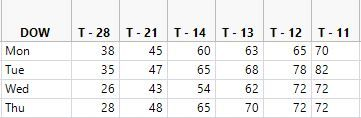1 ACCEPTED SOLUTION

Accepted Solutions
Highlighted

## Re: IF statements basic question

To make the column formula, you can use the if statement or the match statement; the match syntax is a little easier to read:

``````New Table( "example",
New Column( "DOW", character, Set Values( {"Mon", "Tue", "Wed", "Thu"} ) ),
New Column( "T-28", Set Values( [38, 35, 26, 28] ) ),
New Column( "T-21", Set Values( [45, 47, 43, 48] ) ),
New Column( "T-14", Set Values( [60, 65, 54, 65] ) ),
New Column( "T-13", Set Values( [63, 68, 62, 70] ) ),
New Column( "T-12", Set Values( [65, 78, 72, 72] ) ),
New Column( "T-11", Set Values( [70, 82, 72, 72] ) ),
New Column( "Conditional",
Formula(
Match( :DOW,
"Mon", :Name( "T-28" ),
"Tue", :Name( "T-21" ),
"Wed", :Name( "T-14" ),
"Thu", :Name( "T-13" ),
-9999 // else...use . for missing value
)
)
)
)``````

Because the column names contain hyphens (subtract) they need the name() wrapper.

With an if statement:

``````New Table( "example",
New Column( "DOW", character, Set Values( {"Mon", "Tue", "Wed", "Thu"} ) ),
New Column( "T-28", Set Values( [38, 35, 26, 28] ) ),
New Column( "T-21", Set Values( [45, 47, 43, 48] ) ),
New Column( "T-14", Set Values( [60, 65, 54, 65] ) ),
New Column( "T-13", Set Values( [63, 68, 62, 70] ) ),
New Column( "T-12", Set Values( [65, 78, 72, 72] ) ),
New Column( "T-11", Set Values( [70, 82, 72, 72] ) ),
New Column( "Conditional",
Formula(
if(
:DOW=="Mon", :Name( "T-28" ),
:DOW=="Tue", :Name( "T-21" ),
:DOW=="Wed", :Name( "T-14" ),
:DOW=="Thu", :Name( "T-13" ),
-9999 // else...use . for missing value
)
)
)
)``````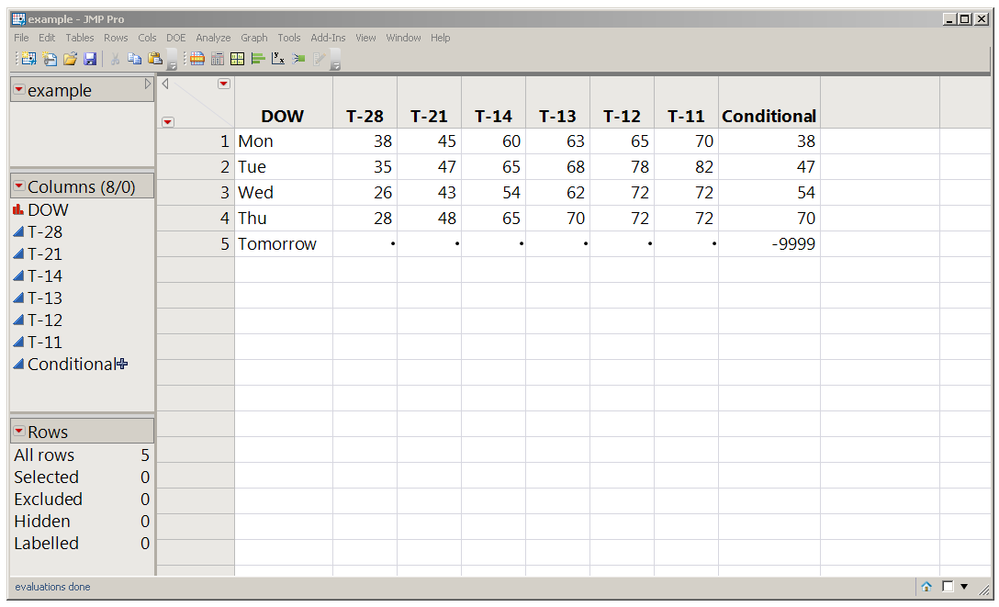The else value handles unknown day names

JMP would really rather use . for a missing value instead of -9999; I used that for this demo so the effect would be clear.

You can also use the Formula Editor instead of JSL; click on the + beside the Conditional column in the data table's left hand panel (above) to see the formula editor (below):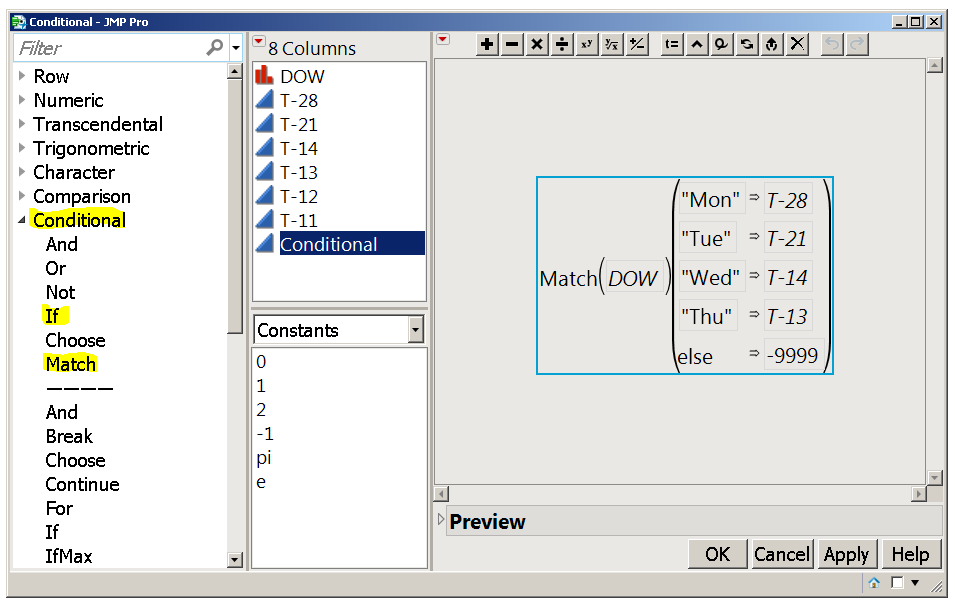The Formula Editor has a list of functions

If Secrets blog post

Craige
3 REPLIES 3
Highlighted

## Re: IF statements basic question

@anjana,
I would advice that you look here. This is a very helpful resource to understand how to use different functions in JMP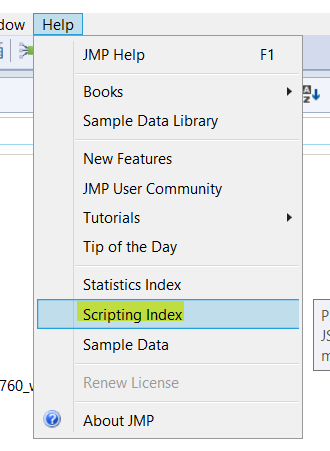Here is an example, which says if x is the value that you are trying to assign to one of the columns, then if the value in column DOW = "Mon", assign the value of x to the column "T-28" , else assign it to column "T-12"

``````If(:DOW == "Mon",
// then  ````  :Name("T-28") = x ;    ,    // else   :Name(T-12) = x;  ); ````
Best
Uday
Highlighted

## Re: IF statements basic question

It is valuable to understand multiple functions. I like to use the power of lists, and prefer not to use functions in my tables, unless I attach a script to set  a column's values.

``````Names Default To Here( 1 );
dt = New Table("example",
New Column("DOW", character, values({"Mon", "Tue", "Wed", "Thu" }) ),
NewColumn("T-28", numeric, values({38,35,26,28})),
NewColumn("T-21", numeric, values({45, 47, 43, 48})),
NewColumn("T-14", numeric, values({60, 65, 54, 65})),
NewColumn("T-13", numeric, values({63, 68, 62, 70})),
NewColumn("T-12", numeric, values({65, 78,72,72})),
NewColumn("T-11", numeric, values({70,82,72,72}))
);

dt << newColumn("Conditional", numeric);

dowlist = {"Mon", "Tue", "Wed", "Thu", "Fri", "Sat"};
clist = {"T-28", "T-21", "T-14", "T-13", "T-12", "T-11"};
dt:Conditional << set each value( Column(dt,clist[Contains(dowlist, :DOW)])[] ) ;``````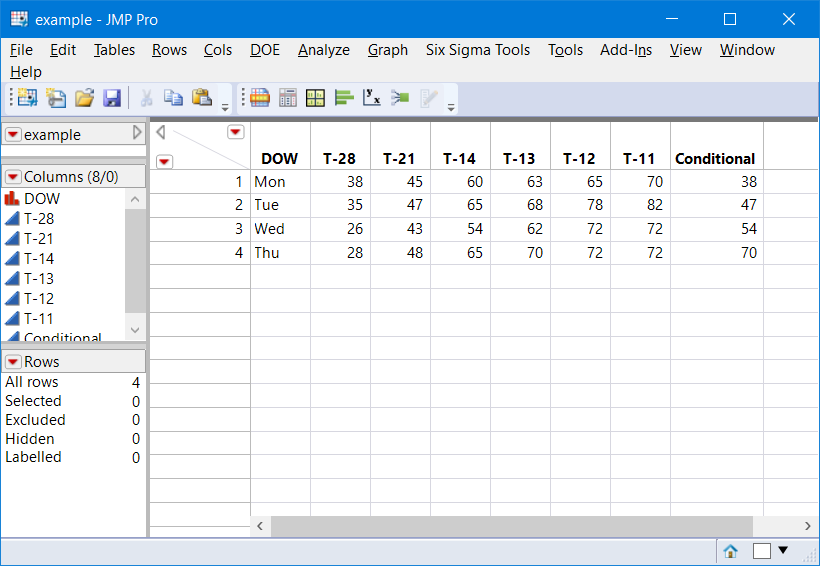Highlighted

## Re: IF statements basic question

To make the column formula, you can use the if statement or the match statement; the match syntax is a little easier to read:

``````New Table( "example",
New Column( "DOW", character, Set Values( {"Mon", "Tue", "Wed", "Thu"} ) ),
New Column( "T-28", Set Values( [38, 35, 26, 28] ) ),
New Column( "T-21", Set Values( [45, 47, 43, 48] ) ),
New Column( "T-14", Set Values( [60, 65, 54, 65] ) ),
New Column( "T-13", Set Values( [63, 68, 62, 70] ) ),
New Column( "T-12", Set Values( [65, 78, 72, 72] ) ),
New Column( "T-11", Set Values( [70, 82, 72, 72] ) ),
New Column( "Conditional",
Formula(
Match( :DOW,
"Mon", :Name( "T-28" ),
"Tue", :Name( "T-21" ),
"Wed", :Name( "T-14" ),
"Thu", :Name( "T-13" ),
-9999 // else...use . for missing value
)
)
)
)``````

Because the column names contain hyphens (subtract) they need the name() wrapper.

With an if statement:

``````New Table( "example",
New Column( "DOW", character, Set Values( {"Mon", "Tue", "Wed", "Thu"} ) ),
New Column( "T-28", Set Values( [38, 35, 26, 28] ) ),
New Column( "T-21", Set Values( [45, 47, 43, 48] ) ),
New Column( "T-14", Set Values( [60, 65, 54, 65] ) ),
New Column( "T-13", Set Values( [63, 68, 62, 70] ) ),
New Column( "T-12", Set Values( [65, 78, 72, 72] ) ),
New Column( "T-11", Set Values( [70, 82, 72, 72] ) ),
New Column( "Conditional",
Formula(
if(
:DOW=="Mon", :Name( "T-28" ),
:DOW=="Tue", :Name( "T-21" ),
:DOW=="Wed", :Name( "T-14" ),
:DOW=="Thu", :Name( "T-13" ),
-9999 // else...use . for missing value
)
)
)
)``````The else value handles unknown day names

JMP would really rather use . for a missing value instead of -9999; I used that for this demo so the effect would be clear.

You can also use the Formula Editor instead of JSL; click on the + beside the Conditional column in the data table's left hand panel (above) to see the formula editor (below):The Formula Editor has a list of functions

If Secrets blog post

Craige
Article Labels

There are no labels assigned to this post.